#Function Repository Resource:

# ChaosGame

Plot iterations for the 2D chaos game

Contributed by: Eric Weisstein
 ResourceFunction["ChaosGame"][n,iter,drop] gives iter iterations of the chaos game on an n-gon with iter points using a ratio of 1/2, dropping the first drop points. ResourceFunction["ChaosGame"][{n,r},iter,drop] uses a ratio r.

## Details and Options

ChaosGame works only in 2D.

## Examples

### Basic Examples

Get three iterations:

 In:=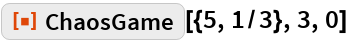Out=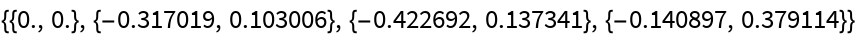Try several geometries:

 In:=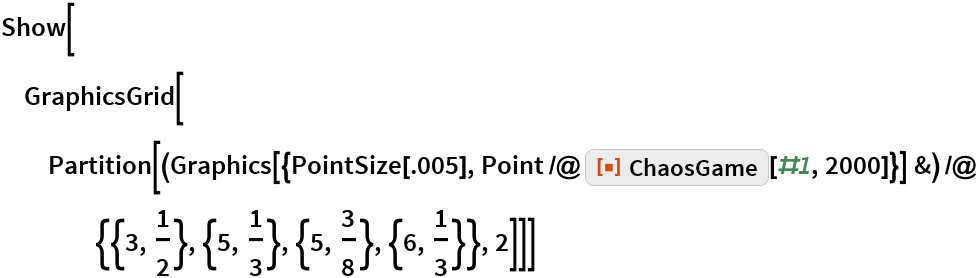Out=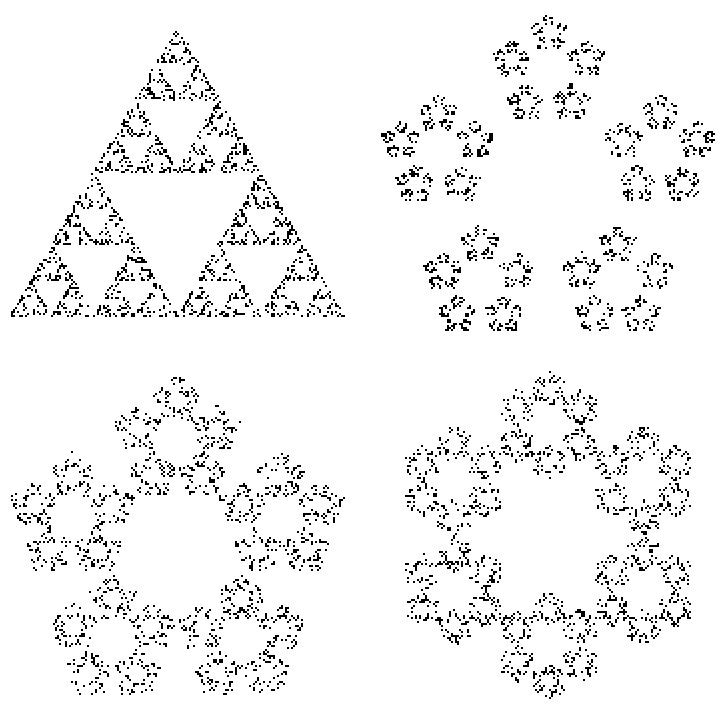With more more iterations:

 In:=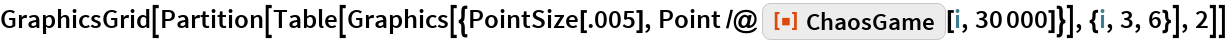Out=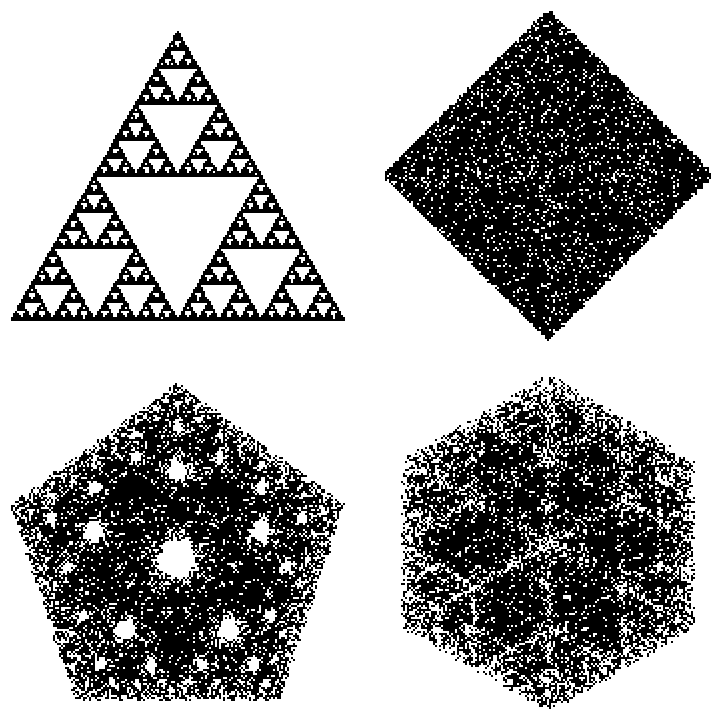Increase ratios:

 In:=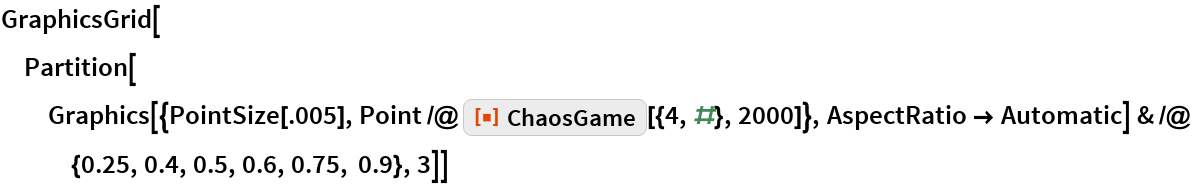Out=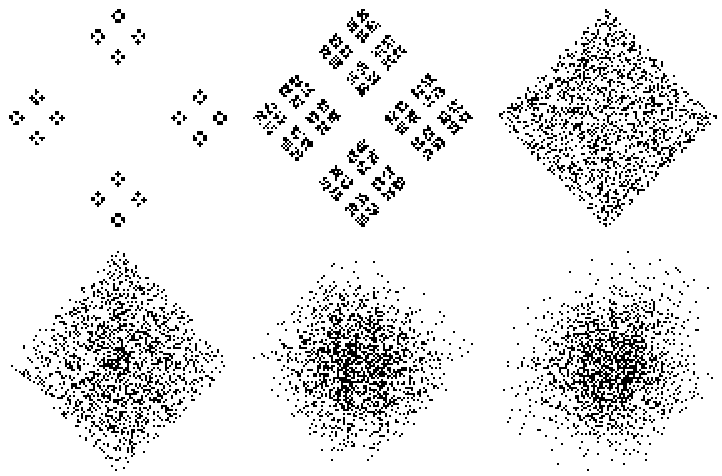An animation of the process:

 In:=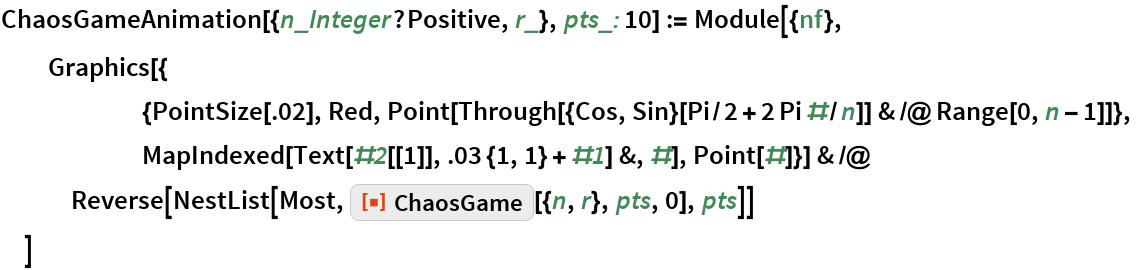In:=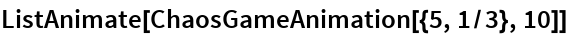Out=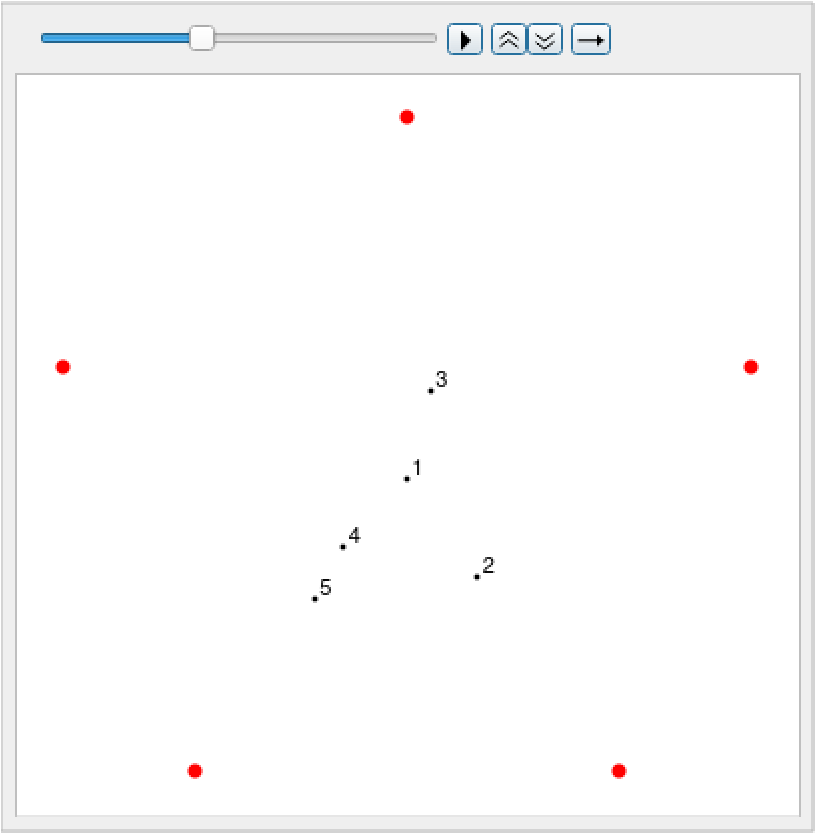## Requirements

Wolfram Language 11.3 (March 2018) or above